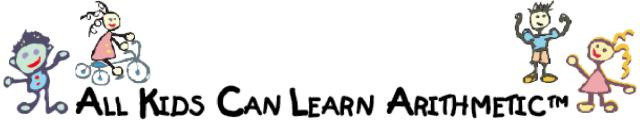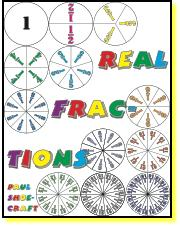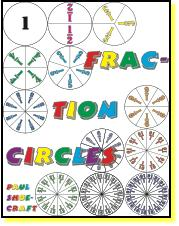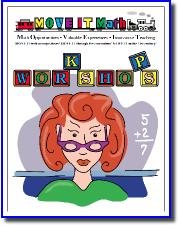MOVE IT Math™ Workshops   •All Kids Can Learn Arithmetic™   •Enrichment & Acceleration   •Advanced Topics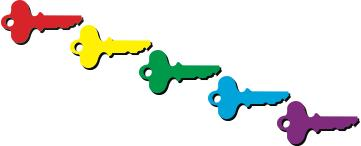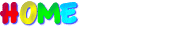Fraction Circles Fraction Circles for Real Fractions: halves, thirds, fourths, fifths, sixths, eighths, ninths, tenths, twelfths, 15ths, 16ths. Three sets: colored, not colored, and blank.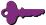Real Fractions Numerators Denominators Comparing Fractions Ordering Fractions Equivalent Fractions Reducing Fractions Adding Fractions Like Denominators Unlike Denominators Subtracting Fractions Like Denominators Unlike Denominators Multiplying Fractions Dividing Fractions